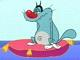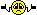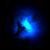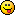Discussion about math, puzzles, games and fun.   Useful symbols: ÷ × ½ √ ∞ ≠ ≤ ≥ ≈ ⇒ ± ∈ Δ θ ∴ ∑ ∫  π  -¹ ² ³ °

You are not logged in.

## #1 2007-11-17 01:52:50

Kurre
MemberRegistered: 2006-07-18
Posts: 280

### Factorization question

When you want to factor a polynom, are there any rules of how to progress? I know the common ones (like a^2+2ab+b^2=(a+b)^2), but i have problems with a bit advanced ones.
for example m^4 - 2m^3 - m^2 + 2m + 1 = 0
can be factored as follows:
(m^2 - m - 1)^2 = 0

It is easy to check that this example is correct, but how do you progress to find the answer? Are there any rules, or do I just need to practice to be able to see the factorization automatically??
really need to get better at this for a math competion next week...
and if you could make a few exercises it would be greatthanks

Offline

## #2 2007-11-17 02:18:35

JaneFairfax
MemberRegistered: 2007-02-23
Posts: 6,868

### Re: Factorization question

Usually we try to find linear factors. For example, if you suspect that (x−3) is a factor, you substitute x = 3 and see if it makes the polynomial 0. For nonlinear factors, things are just too complicated. Even if you temporarily allow complex factors, things are still complicated. Sorry, I dont know any general method to factorize polynomials into nonlinear factors.Offline

## #3 2007-11-17 02:41:10

Identity
MemberRegistered: 2007-04-18
Posts: 934

### Re: Factorization question

Try the rational root theorem to find potential rational roots (and use them as Jane suggested). If none of them work, then there are no rational linear factors and it will be truly hard to factorise. Either it will have all non-linear factors, or have irrational factors.
It goes like this: For any polynomial

with integer coefficients, all rational roots will be of the form

, where |p| and |q| are relatively prime integers, p divides
evenly, and q divides
evenly.
As you might see now, it gets hard if
and
have lots of factors.

Example:

Factorise:

Possible values of p and q are:

So the possible values of p/q are:

Trying the factor theorem with x = 1,

Therefore, (x-1) is a factor. Dividing this into the polynomial you get the remaining factors:

Last edited by Identity (2007-11-17 03:10:30)

Offline

## #4 2007-11-17 02:49:41

Identity
MemberRegistered: 2007-04-18
Posts: 934

### Re: Factorization question

Try these exercises (all can be solved with the rational root theorem but maybe you can find a quicker way):

Factor:

If you get factorisation problems with more than 1 pronumeral, as in example 3, you can still use the method but be careful that sometimes x may have no linear factors but y for instance will. In that case try to put the polynomial in terms of y and think of x as a constant.

The following problem comes from some sort of competition. It's a very, very hard oneFactor the following:

Last edited by Identity (2007-11-17 03:34:24)

Offline

## #5 2007-11-17 03:52:51

JaneFairfax
MemberRegistered: 2007-02-23
Posts: 6,868

### Re: Factorization question

Factorizing into linear factors is generally easy. I think Kurre is after more complicated nonlinear factorizations. For example:

And, Identity:

Last edited by JaneFairfax (2007-11-17 04:22:13)

Offline

## #6 2007-11-17 23:43:58

Kurre
MemberRegistered: 2006-07-18
Posts: 280

### Re: Factorization question

Thanks a lot for the tips, they sure did help!
and thanks for the exercises Identity
just a  fast question, in ex. 4 i can only find the factor x-3, is that the only one?

Offline